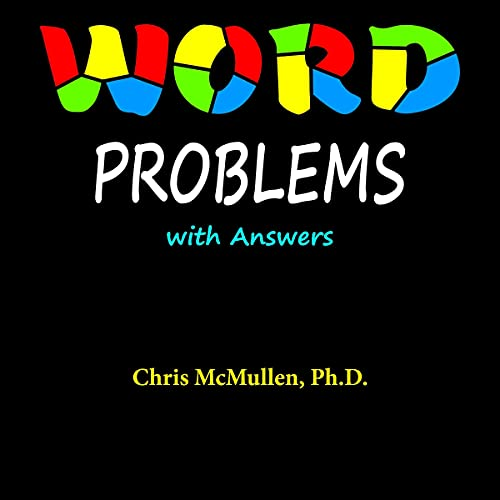Non-member price: \$27.79

After 30 days, Audible is \$16.45/mo. Cancel anytime.

## Publisher's Summary

This book includes 200 word problems with answers. Some tips and a few examples are also provided. Several topics are included, such as:

• 11 age problems
• 15 rate problems
• Three mixture problems that do not involve liquids (these more tangible mixtures involve rocks or balls, which allow students to develop an understanding of how the mixture concept works)
• Four problems involving working together (or against)
• Four problems that are similar to the classic handshaking problem
• Several problems that involve ratios or proportions
• Seven problems that involve counting permutations or combinations
• 10 problems that involve calculating the likelihood of an event occurring
• Several problems that feature geometric shapes
• Four problems that require making predictions
• Three problems that involve other number systems, like base three or nine
• Several counting problems
• 10 comparison problems
• Five remainder problems
• Several problems that involve money
• Several problems that involve fractions, decimals, or percentages
• And a variety of other word problems

The author, Chris McMullen, PhD, has over 20 years of experience teaching math skills to physics students. He prepared this workbook of the Improve Your Math Fluency series to share his passion for word problems.

©2020 Chris McMullen (P)2021 Chris McMullen